# Find Duplicate Elements in an Array using Hashing in C

## Finding Duplicate Elements in a Sorted Array using Hashing in C:

In this article, I am going to discuss Finding Duplicate Elements in a Sorted Array using Hashing in C Language with Examples. In our previous article, we have seen how to find duplicate elements in a sorted array.

##### How to Find Duplicate Elements in a Sorted Array using Hashing in C Language?

We have the following sorted array.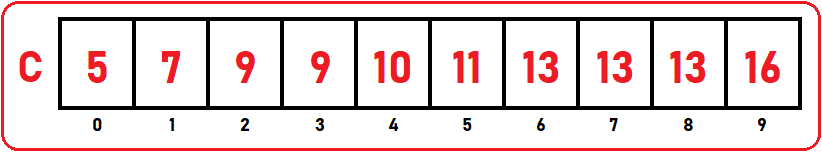We have taken a sorted array that has duplicated elements. As we can see elements reoccur one or more than one time in the above array. We want to know the duplicate elements as well as their count i.e. 13 is duplicated, then how many times it duplicated. For this, we are using a method that will use a hash table. As we have seen in the hash table in our previous articles. Below is the simplest form of hast table: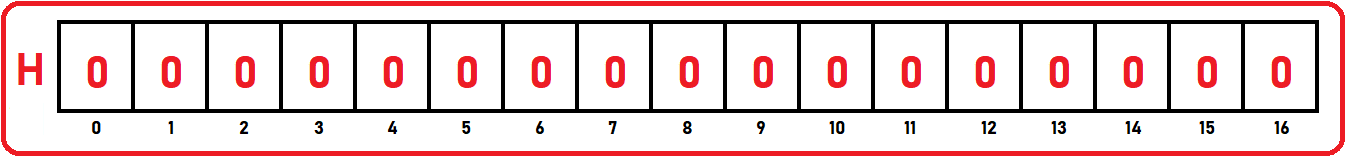We take the size of hast table 16 because 16 is the largest element in the above-given array. As we have taken a sorted array so we can find the largest element at the end of the array. Next, we have to fill the hash table or extra array with zeroes. Now, let’s look at the procedure:

We have to scan through the given array by taking one element at a time.

##### 0th Index:

We are taking an index pointer ‘k’ which is starting from the 0th index: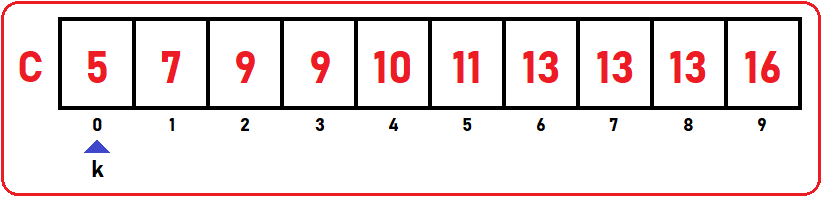k is at C  which is 5. So go to the extra array and increment the value of index 5: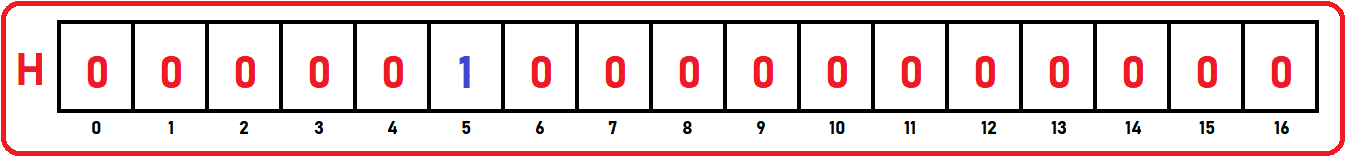##### 1st Index:

Now, ‘k’ is pointing to the 1st index: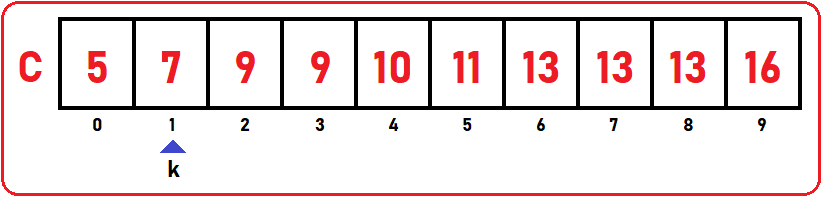k is at C  which is 7. So go to the extra array and increment the value of index 7: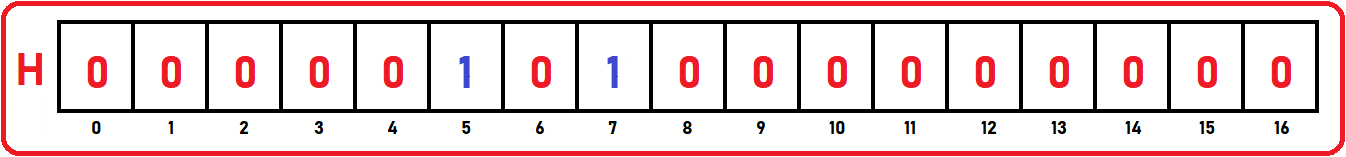##### 2nd Index:

Now, ‘k’ is pointing to the 2nd index: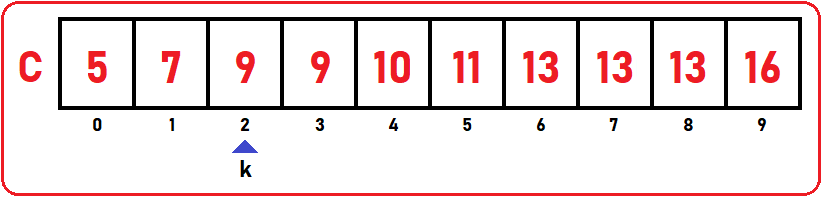k is at C  which is 9. So go to the extra array and increment the value of index 9: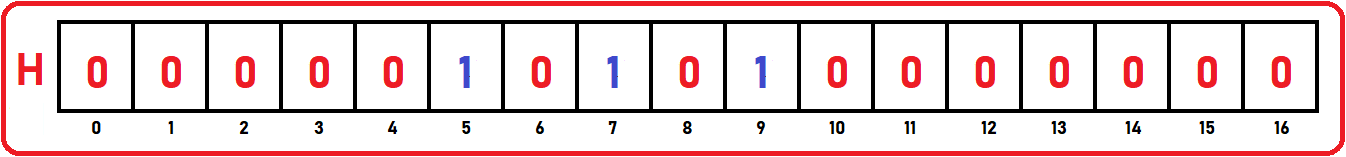##### 3rd Index:

Now, ‘k’ is pointing to the 3rd index: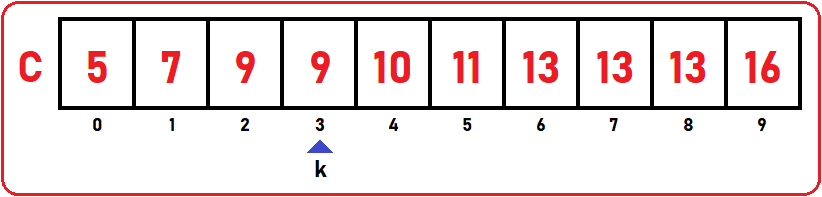k is at C  which is 9 again. In the extra array, increment the value of index 9: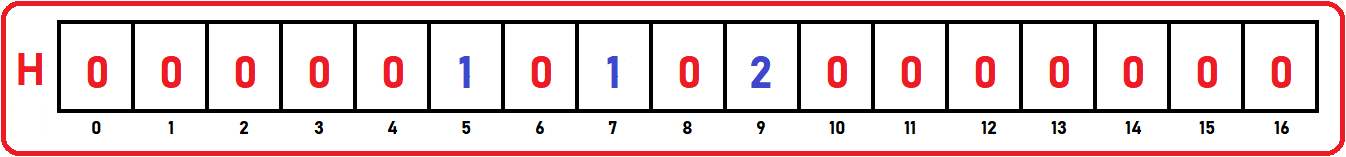In the same manner, we will trace the whole array and increment the value of the extra array as we will find duplicate elements. So, at last, our extra array will be: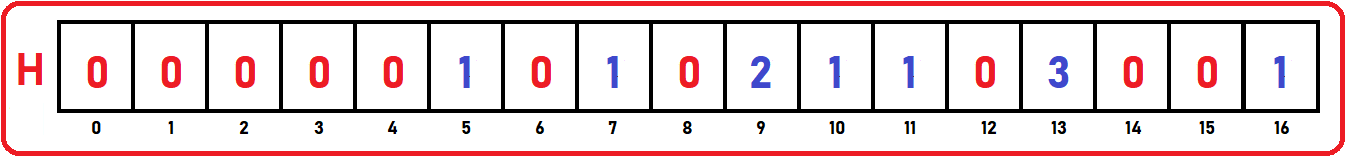So, when we will find any duplicate element then we will increment the value of the same index number in the extra array ‘H’.

Let’s do some analysis. So, how many times we are scanning through the array? Only 1 time. While scanning we are incrementing the count in the extra array. So, it will be constant time. The major time we spent is O (n). Now we have to find out what are the duplicate elements from the above hast table. So, we will scan for 1. For scanning the hash table, it will take O (n). Here n means linear.

##### Full Code in C language:
```#include <stdio.h>
#include <stdlib.h>

struct List{
int C;
int size;
int length;
};

void Display(struct List list) {
int i;
printf("Elements are:\n");
for (i = 0;i<list.length;i++)
printf("%d ", list.C[i]);
printf("\n\n");
}

void DuplicateHashTable(struct List list, int max){

int H[max+1];

for(int i = 0; i <= max; i++){
H[i] = 0;
}

for(int i = 0; i < list.length; i++){
H[list.C[i]]++;
}

printf("Duplicate Elements are: \n");
for (int i = 0; i <= max; i++){
if(H[i] > 1)
printf("%d is appearing: %d times\n", i, H[i]);
}
}

int main(){
struct List list_1 = {{5, 7, 9, 9, 10, 11, 13, 13, 13, 16}, 10, 10};

Display(list_1);

DuplicateHashTable(list_1, 16);
}
```
###### Output: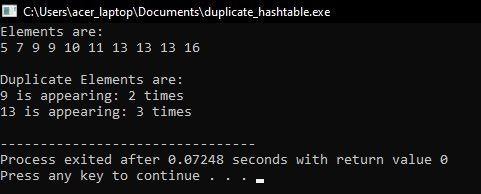If we have a single for loop then time will be n and if we have for loop inside for loop then it will take n2.

Time Complexity: O(n)

In the next article, I am going to discuss How to Find Duplicate Elements in an Unsorted Array in C Language with Examples. Here, in this article, I try to explain How to Find Duplicate Elements in a Sorted Array using Hashing in C Language with Examples and I hope you enjoy this Finding Duplicate Elements in a Sorted Array using Hashing in C Language with Examples article.